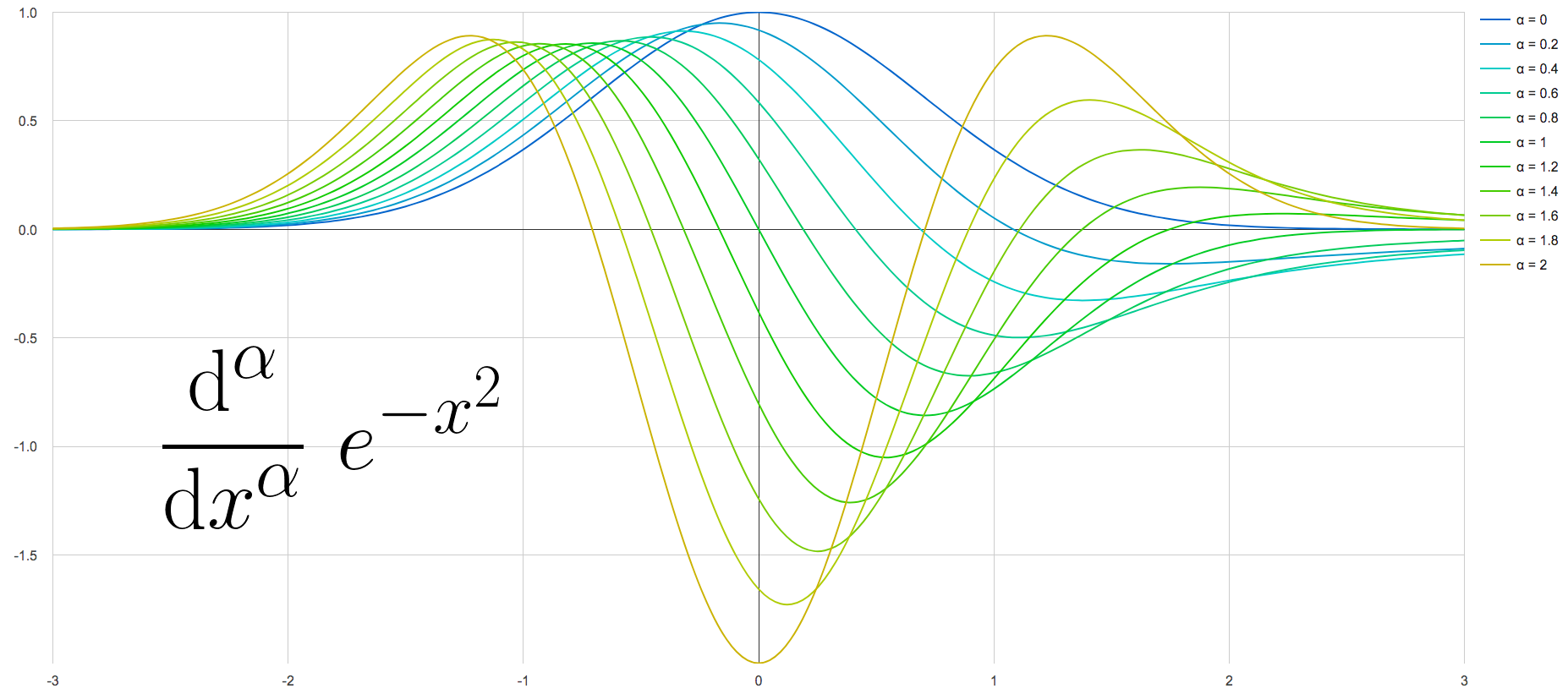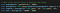Fractional derivatives of exp(-x*x), starting with original function (order 0, blue) all the way to second derivative (order 2, yellow) in steps of 0.2

# Interactive Fractional Derivative Playground

## Real-time visuals for everyone

This is just a short story about the online Fractional Derivative Playground that I created out of curiosity and wanted to share with other curious minds. Disclaimer: I am not a mathematician, so take everything I claim or the playground shows with a grain of salt.

TL;DR: Fractional derivatives are a thing; I couldn’t find any nice interactive online visualization; I made one; let me know what you think!

One day my YouTube feed contained Dr. Peyam’s Definition of the Half Derivative. I didn’t even know something like that exists, must watch.

Dr. Peyam made my day. Derivatives and integrals had a clear place in my head, a certain intuition and familiarity from back in school. But what I saw here added a whole new dimension. The idea made sense, the examples worked out, but what about intuition? It’s no longer just the slope of or area under a curve.

So you learn about this formula to compute a fractional derivative of order α and about a few examples of special functions (like sin(x) or x^n) where the formula simplifies nicely. But what do other functions look like? I don’t want to spend ages reducing a formula for some specific α, hoping for it to click in my head. Also, symbolic integration gets very hard very quickly. Numerical integration on the other hand is what PCs are good for!

How about building an intuition about fractional derivatives without some nice plots? The closest thing I could find was this gif for f(x)=x. I wanted a website plotting the order α derivative of f, letting you specify f and α.large plot area constantly showing f and f’ (dashed) as well as a fractional derivative specified by you

I hacked together approximations of both Riemann–Liouville derivative and Grünwald–Letnikov derivative. Note that the GUI let’s you choose the algorithm used via the drop-down at the bottom. Sources of inaccuracy in my approximations:

• Riemann–Liouville involves approximating an integral (summing up lots of tiny trapezoids) and then a derivative of that (using some magically chosen small numbers).
• Grünwald–Letnikov involves approximating an infinite sum (so hope that it converges reasonably fast and stop at some point) and the same kind of limit as above, i.e. a small magic number. Conceptually simpler IMO.

This website is a big hack. Here is a taste of the guesswork happening:non-scientific test for divergence followed by non-scientific choice of magic numbers

There is scientific work about quickly evaluating fractional derivatives with reasonable error and such. That would be a next step. I don’t even know how wrong my approximations are (you can sometimes observe slight jumps when switching between the two algorithms). My initial goal of having a tool to build intuition is achieved. But you are more than welcome to contribute!

compiler and programming language enthusiast

compiler and programming language enthusiast